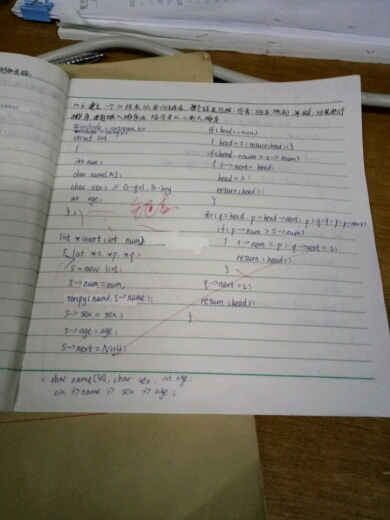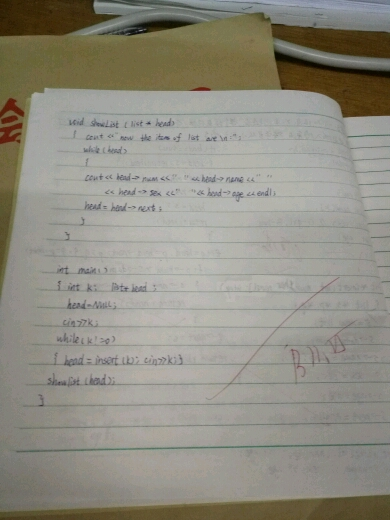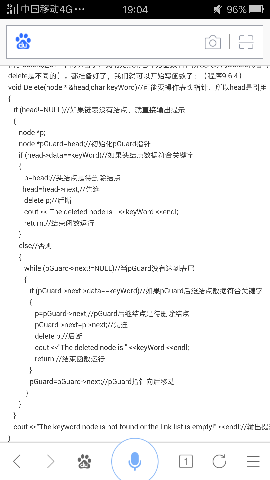2015-12-21 10:43

# C++链表（我写的程序自己也看不懂）

（我链表没听懂，基础概念讲讲也好~）

• 点赞
• 写回答
• 关注问题
• 收藏
• 邀请回答

#### 10条回答默认 最新

•John_ToString 2015-12-22 02:12
已采纳

以下是创建链表的代码，c语言结构体实现：(不带头结点，一级指针实现，当然可以多级指针实现，也可以带头结点，也可以是循环链表，也可以是
双向循环链表)
#include
#include
//链表结构体定义
typedef struct _NODE
{
int data;
struct _NODE *next;
}Node, *List;

//创建链表
List CreateList()
{
Node head = NULL, *p1 = NULL, *p2 = NULL;
p1 = p2 = (Node
)malloc(sizeof(Node));

``````            while(1 == scanf("%d", &p1->data))
{
{
p1->next = NULL;
}
else
{
p2->next = p1;
p2 = p1;
}
p1 = (Node*)malloc(sizeof(Node));
}
free(p1);
p1 = NULL;
p2->next = NULL;
/*注意这里 若果是 p2->next = p1; 那么就成为了一个单向循环链表*/
``````

}

点赞 评论
•陌湘萘 2015-12-21 10:47

我的程序……少建立链表了，怎么建呢？
网上有些链表的程序里.data什么的是默认的参数（数据成员）吗？

点赞 评论
•老师讲一遍你都不耐烦听，写一遍给你你更不耐烦看了。

单向链表简单来说就是一个数据结构体，每个节点包括自身的数据和下一个节点的指针。因此，你可以像链子一样遍历得到它。至于插入，很容易理解，就是先记录下原先那个节点指向的后继节点的地址，让它指向新的节点，而新的节点再指向原先那个节点指向的节点的地址。
数据结构书上一般会有图，看下就懂了。如果没图，去图书馆借一本好一点的书。

点赞 评论
•陌湘萘 2015-12-21 10:55

我好像没建立链表，怎么改？点赞 评论
•陌湘萘 2015-12-21 11:09

问的另一个问题:举个例子，（如图）这里->data是之前定义过的吗？
它所代表的数据是指链表中的某一小段中的除去 头 和next的那些吗？还是特指叫作data的一小段？点赞 评论
•陌湘萘 2015-12-21 11:36

{
{
node *p;
{
delete p;
cout <<"The deleted node is " <          return;
}
else
{
while (pGuard->next!=NULL)
{
if (pGuard->next->data==keyWord)//这里为什么会有两个→
{
p=pGuard->next;
pGuard->next=p->next;
delete p;
cout <<"The deleted node is " <                return;
}
pGuard=pGuard->next;
}
}
}
}

这段代码是网上找的，整个程序我无法见到，所以有点盲人摸象的意思……
我想知道data是定义类或结构体中已经定义过的成员吗？函数中用到的只是对应的名为data的那部分吗？
还是说data是特定的系统定义的概念似的某一部分？它所对应的是引用的成员中去掉next的剩下的东西吗？
我还想知道data实际实现过程中是怎样变化的？

点赞 评论
•Svvvvvm 2015-12-21 12:28

typedef struct LNode{ // 定义单链表节点类型

ElemType data;

LNode *next; // 指示下一个结点地址的指针
} LNode;

void InitList (LNode *&H) //初始化单链表
{ //构造一个空的线性链表H，即为链表设置一个头结点，
//头结点的data数据域不赋任何值，头结点的指针域next则为空
H=new LNode; //(LNode *)malloc(sizeof(LNode));

if (!H)
exit(0);
H->next=NULL; // 指针域为空
}
void ClearList(LNode *&H) //清除单链表
{
LNode *q=H->next;
while (q!=NULL) {
H->next =q->next;
free(q);
q=H->next;
}
}

int LengthList (LNode *H) //求单链表长度
{
LNode *p=H;int n=0;
while(p->next!=NULL){
n++;
p=p->next; }
return(n);
}

bool EmptyList (LNode *H) //判断单链表是否为空表
{
return(H->next==NULL);
}

ElemType GetList (LNode *H, int pos) //取单链表第 pos 位置上的元素
{
int j=0;
LNode *p=H;
while(j j++;
p=p->next;
}
if(p==NULL) return 0;
else {
return(p->data);
}
}

void TraverseList(LNode *H) //遍历单链表
{
LNode *p=H->next;
while(p!=NULL){
printf("%d",p->data);
p=p->next;
}
printf("\n"); }

bool InsertList ( LNode *&H, ElemType item, int pos) //向单链表插入一个元素
{
int j=0;
LNode *p=H,*s;
while(j j++;
p=p->next; }
if(p==NULL) return 0;
else {
s=(LNode *)malloc(sizeof(LNode));
s->data=item;
s->next=p->next;
p->next=s;
return 1;
}
}

bool DeleteList ( LNode *&H, ElemType &item, int pos) //从单链表中删除一个元素
{
int j=0;
LNode *p=H,*q;
while(j j++;
p=p->next; }
if(p==NULL)
return 0;
else {
q=p->next;
if(q==NULL)
return 0;
item=q->data;

p->next=q->next;
free(q);
return 1;
} }

``````————————————————————————————————LinkList.h————————————

#include <stdio.h>
``````

#include
#include
typedef int ElemType;

int main() {

LNode *h;

ElemType e;

InitList(h); //1

cout<<"请输入要插入元素的个数:";

int length;

cin>>length;
if(length == 0)

cout<<"链表长度为0.";

``````    else{
cout<<"输入数据:";
for(int i = 0; i < length; i++)  {
int t;
cin>>t;
InsertList(h,t,i+1);
}
TraverseList(h); //6
}

if(EmptyList(h))  //4
cout<<"单链表h为空"<<endl;
else
cout<<"单链表h非空"<<endl;
int m;
m=LengthList(h);
cout<<"单链表h长度= "<<m<<endl;    //3

cout<<"请输入要查找的元素的位置:";//5
int f;
cin>>f;
if(f>m||f<1){
cerr<<"pos is out of range!"<<endl;
return 0;  }
else{
e=GetList(h, f);
cout<<"元素="<<e<<endl;
}
cout<<"请输入要插入元素的位置:";  //7
int pos;
cin>>pos;
if(pos>length){
cerr<<"pos is out of range!"<<endl;
return 0;  }

cout<<"请输入要插入的元素:";
int x;
cin>>x;
InsertList(h, x, pos);
printf("输出单链表h:");
TraverseList(h);
cout<<"请输入要删除元素位置:"; //8
int y;
cin>>y;
DeleteList(h, e, y);

TraverseList(h);

ClearList(h);//2
return 0;
``````

}

————————————————————————————————————test2_2.cpp————————

``````        以前写的。希望对你有帮助。
``````
点赞 评论
•John_ToString 2015-12-22 02:14

补充一下，你是初学者，.data不是什么默认参数，是用户自定义的，你可以定义为你自己喜欢的任意单词。比如说student结构体里面的age（年龄）...

点赞 评论
•John_ToString 2015-12-22 02:15